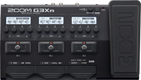# metal

Discussion in 'Zoom G3n/G3Xn/G5n' started by Josh Strope, Dec 2, 2019.

1. metalDevice: Zoom G3Xn
Firmware: 2.20

Name on device: metal1
Optimized for: Guitar Amp

Effects chain:dope

Effect: "ZNR" (Dynamics), active - "yes"
"Detect" = EFXIN
"Depth" = 100
"Threshold" = 30
"Decay" = 0

Effect: "Gt GEQ" (Filter), active - "yes"
"160 Hz" = 0.0
"400 Hz" = 0.0
"800 Hz" = 0.0
"3.2 kHz" = 0.0
"6.4 kHz" = 0.0
"12.0 kHz" = 0.0
"Volume" = 80
"CH SEL" = UnLATCH
"160 Hz" = 0.0
"400 Hz" = 0.0
"800 Hz" = 0.0
"3.2 kHz" = 0.0
"6.4 kHz" = 0.0
"12.0 kHz" = 12.0
"Volume" = 0
"CH SEL" = UnLATCH
"Parameter set" = A

Effect: "MetalWRLD" (Overdrive / Distortion), active - "yes"
"Gain" = 60
"Bass" = 50
"Treble" = 50
"Volume" = 80

Effect: "MS4x12" (Cabinet), active - "yes"
"MIC" = ON
"D57: D421" = 50
"Hi" = 50
"Lo" = 51

Effect: "Rhythm" (Rhythm and Looper), active - "yes"
"Pattern" = MtlCore 4/4
"Unknown1" = 0
"Unknown2" = 0
"Volume" = 80

Patch Volume: 100